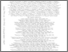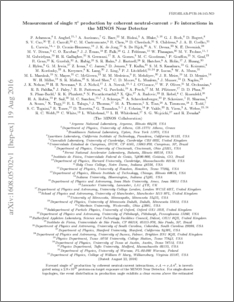# Measurement of single $π^0$ production by coherent neutral-current $ν$ Fe interactions in the MINOS Near Detector

, MINOS COLLABORATION (2016) Measurement of single $π^0$ production by coherent neutral-current $ν$ Fe interactions in the MINOS Near Detector. Physical Review D, 94 (7). ISSN 1550-7998Preview
PDF (1608.05702v1)
1608.05702v1.pdf - Accepted Version

## Abstract

Forward single $\pi^0$ production by coherent neutral-current interactions, $\nu \mathcal{A} \to \nu \mathcal{A} \pi^0$, is investigated using a 2.8$\times 10^{20}$ protons-on-target exposure of the MINOS Near Detector. For single-shower topologies, the event distribution in production angle exhibits a clear excess above the estimated background at very forward angles for visible energy in the range~1-8 GeV. Cross sections are obtained for the detector medium comprised of 80% iron and 20% carbon nuclei with $\langle \mathcal{A} \rangle = 48$, the highest-$\langle \mathcal{A} \rangle$ target used to date in the study of this coherent reaction. The total cross section for coherent neutral-current single-$\pi^0$ production initiated by the $\nu_\mu$ flux of the NuMI low-energy beam with mean (mode) $E_{\nu}$ of 4.9 GeV (3.0 GeV), is $77.6\pm5.0\,(\text{stat}) ^{+15.0}_{-16.8}\,(\text{syst})\times10^{-40}\,\text{cm}^2~\text{per nucleus}$. The results are in good agreement with predictions of the Berger-Sehgal model.

Item Type:
Journal Article
Journal or Publication Title:
Physical Review D